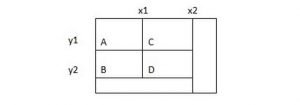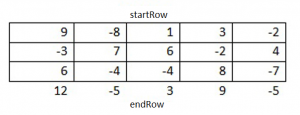# How to Find the Submatrix Sum

The essence of the problem that this test had was to find the submatrix sum of the elements. After a short period of time, our expert solved this problem. The challenge was easily accepted by our expert and now you can see the excellent result of it. Despite the fact that our sample can help students with their homework, we can offer an even better solution. AssignmentShark.com can find solutions to any of your homework problems. The very idea of our service is to provide math assignment help students with technical tasks in the shortest period of time.

First, you need to place your order on our site mentioning your requirements and setting the deadline. Obviously, we try to make the order system simple so you will not be confused. If you receive a completed order from us and don’t like something in it, you can request free revisions. We work 24/7 to meet “do my homework for me free” requests. Check out our sample and find some helpful ideas to deal with your own homework.

## Submatrix With the Largest Sum of Elements

We have the matrix N*N containing positive and negative numbers. We need to find the submatrix with the largest sum of elements.

There are many solutions to this problem. We will start with the most obvious method, then we will optimize it.

## The most obvious method: O(N6)

Like the other tasks associated with the search of the maximum, this problem has a simple solution. It is enough to check all submatrices, calculate the sum of each, and to find the largest one.

To check out all the submatrices and avoid duplication we will have to go through all the ordered pairs of rows and then all ordered pairs of columns.

This solution will require O(N) time, since it is necessary to check the O(N) matrices, and the check of one matrix takes O(N²) time.

## Dynamic programming: O(N⁴)

Note that the previous solution is slow due to the calculation of the sum of matrix elements – O(N²) is a very slow operation. We can reduce the time of calcSum to O(1).

Take a look at the following rectangle:Assume that we know the following values:

ValA = area(point(xl, yl))

ValB = area(point(xl, y2))

ValC = area(point(x2, yl))

ValD = area(point(x2, y2))

Each of these values begins at the starting point and ends at the bottom right corner of the subrectangle. We know the following about these values:

area(D) = D – area(A and С) – area(A and B) + area(A).

or

area(D) = D – B – C + A

This information will let us effectively calculate these values for all points of the matrix:

Уа1(х, у) = Уа1(х-1, у) + Уа1(у-1, х) – Уа1(х-1, у-1)

We can calculate these values and then find the maximal submatrix. The following code implements this algorithm:

```int maxMatrix(int[][] inputMatrix) {
int maximalSum = Integer.MIN_VALUE; // Maximal sum can be negative
int rows = inputMatrix.length;
int cols = inputMatrix.length;
int[][] matrix = precalcMatrix(inputMatrix);
for (int rowl = 0; rowl < rows; rowl++) {
for (int row2 = rowl; row2 < rows; row2++) {
for (int coli = 0; coli < cols; coll++) {
for (int col2 = coli; col2 < cols; col2++) {
maximalSum = Math.max(maximalSum, calcSum(matrix, rowl, row2, coli, col2));
}
}
}
}
return maximalSum
}

int[][] precalcMatrix(int[][] matrix) {
int[][] sumMatrix = new int[matrix.length][matrix.length];
for (int i = 0; i < matrix.length; i++) {
for (int j = 0; j < matrix.length; j++) {
if (i == 0 && j == 0) { // first cell
sumMatrix[i][j] = matrix[i][j];
} else if (j == 0) { // cell in the first column
sumMatrix[i][j] = sumMatrix[i - l][j] + matrix[i][j];
} else if (i == 0) { // cell in the first row
sumMatrix[i][j] = sumMatrix[i][j-1] + matrix[i][j];
} else {
sumMatrix[i][j] = sumMatrix[i-l][j] +
sumMatrix[i][j-1] - sumMatrix[i-l][j-1] +
matrix[i][j];
}
}
}
return sumMatrix;
}

int calcSum(int[][] sumMatrix, int il, int i2, int jl, int j2) {
if (il == 0 && jl == 0) { // row 0, column 0
return sumMatrix[i2][j2];
} else if (il == 0) { // row 0
return sumMatrix[i2][j2] - sumMatrix[i2][jl - 1];
} else if (jl == 0) { // column 0
return sumMatrix[i2][j2] - sumMatrix[il-l][j2];
} else {
return sumMatrix[i2][j2] - sumMatrix[i2][jl-1] - sumMatrix[il-l][j2] + sumMatrix[il-1][jl-1];
}
}

```

## Optimized solution: O(N³)

There is a better solution. If we have R rows and C columns, the problem can be solved in O (R²C) time.

Each submatrix can be represented as a series of rows and columns. You can walk along the rows and columns to find what is giving the maximum amount.

The code looks like this:

max = 0

foreach startRow in rows

foreach endRow in rows

max = max(currentMax, max)

return max

Now we have a question: how to find the “best” startCol and endCol?

Let’s take a look at the submatrix:We need to find startCol and endCol, which will give us the biggest possible sum of all submatrices startRow at the top and endRow at the bottom. We can calculate the sum of each column and use the maxSubArr function.

Now we can write the following pseudocode:

max = 0

foreach startRow in rows

foreach endRow in rows

foreach col in columns

fractionalSum[col] = sum of matrix[startRow, col] through

matrix[endRow, col]

currentMax = maxSubArray(fractionalSum)

max = max(currentMax, max)

return max

Calculation of the sum in rows 5 and 6 takes R*C time which gives the total time O(R³C).

max = 0

foreach startRow in rows

clear array fractionalSum

foreach endRow in rows

foreach col in columns

fractionalSum[col] += matrix[endRow, col]

currentMax = maxSubArray(fractionalSum)

max = max(currentMax, max)

return max

The full version of the code looks like this:

```public void clear(int[] arr) {
for (int i = 0; i < arr.length; i++) {
arr[i] = 0;
}
}

public static int maxSubMatrix(int[][] originalMatrix) {
int rows = originalMatrix.length;
int cols = originalMatrix.length;

int[] fractionalSum = new int[cols];
int max = 0;

for (int startRow = 0; startRow < rows; startRow++) {
clear(fractionalSum);

for (int endRow = startRow; endRow < rows; endRow++) {
for (int i = 0; i < cols; i++) {
fractionalSum[i] += originalMatrix[endRow][i]j
}

int tempMaxSum = maxSubArray(fractionalSum, cols);

max = Math.max(max, tempMaxSum);
}
}
return max;
}

public static int maxSubArray(int arr[], int N) {
int max = 0;
int currentSum = 0;

for (int i = 0; i < N; i++) {
currentSum += arr[i];
max = Math.max(max, currentSum);

if  (currentSum < 0) {
currentSum  = 0;
}
}
return  max;
}

```

This is an extremely difficult task. But as we can see, nothing is impossible!